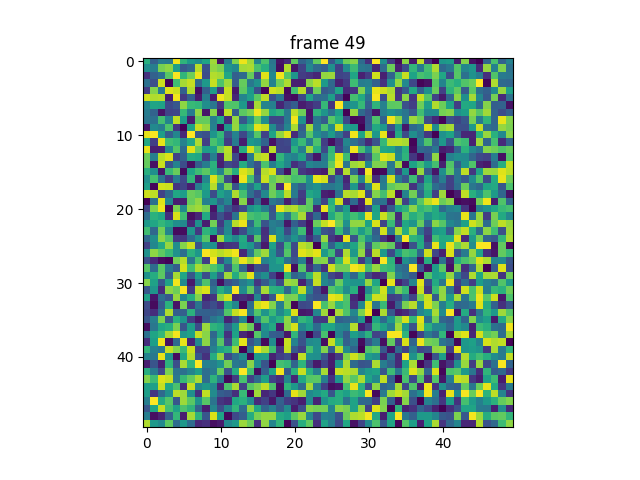# pyplot animation¶

Generating an animation by calling pause between plotting commands.

The method shown here is only suitable for simple, low-performance use. For more demanding applications, look at the animation module and the examples that use it.

Note that calling time.sleep instead of pause would not work.import matplotlib.pyplot as plt
import numpy as np

np.random.seed(19680801)
data = np.random.random((50, 50, 50))

fig, ax = plt.subplots()

for i in range(len(data)):
ax.cla()
ax.imshow(data[i])
ax.set_title("frame {}".format(i))
# Note that using time.sleep does *not* work here!
plt.pause(0.1)


Total running time of the script: ( 0 minutes 8.024 seconds)

Keywords: matplotlib code example, codex, python plot, pyplot Gallery generated by Sphinx-Gallery